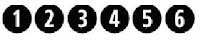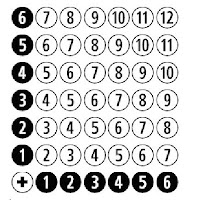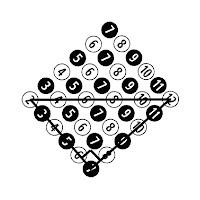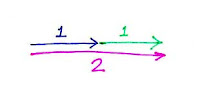## Tuesday, February 21, 2012

### Why is the SD of the sum proportional to the square root?

(Warning: Math/teaching statistics post. No sports this time.)

----

If you take the sum of two identical independent variables, the SD of the sum is *not* two times the SD of each variable. It’s only *the square root of two* times the SD.

There’s a mathematical proof of that, but I’ve always wondered if there was an intuitive understanding of why that is.

First, you can get a range without doing any math at all.

It seems obvious that when you add up two variables, you’re going to get a wider spread than when you just look at one. For instance, you can see that it’s easier to go (say) 10 games over .500 over two years, than over just one year. You can see that if you roll one die, it’s hard to go two points over or under the average (you have to roll a 1 or 6). But if you roll 100 dice, it’s easier to go two points over the average (You can roll anything except 349, 350, or 351.)

So, the spread of the sum is wider than the spread of the original. In other words, it's more than 1.00 times the original.

Now, if you just doubled everything, it’s obvious that the multiplier would be 2.00, that the curve would be twice as wide. A team that goes +10 over one season will go +20 over two seasons. The team that goes -4 will go -8. And so on. So, if you do that, it's exactly 2.00 times the original.

But, in real life, you don't just double everything -- there’s regression to the mean. The team that goes +10 one random season probably will go a lot less than +10 the second random season. And if you roll 6 on the first die, you're probably going to roll less than 6 the second die. So the curve will be less stretched out than if you just doubled everything.

That means the multiplier has to be less than 2.00.

So, the answer has to be something between 1.00 and 2.00. The square root of two is
1.41, which fits right in. It seems reasonable. But why exactly the square root of two? Why not 1.5, or 1.3, or 1.76, or Pi divided by 2?

I’m looking for an intuitive way to explain why it’s the square root of two. I’ve come up with two different ways, but I’m not really happy with either. They’re both ways in which you can see how the square root comes into it, but I don’t think you really *feel* it.

Here they are anyway. Let me know if you have improvements, or you know of any others. I’m not looking for a mathematical proof -- there are lots of those around -- I’m just looking for an explanation that lets you say, “ah, I get it!”

-------

Explanation 1:

First, I’m going to cheat a bit and use something simpler than a normal distribution. I’m going to use a normal six-sided die. That’s because of my limited graphics skills.

So, here’s the distribution of a single die. Think of it as a bar graph, but using balls instead of bars.Part of my cheating is that I’m going to use the shortcut that the SD of a distribution is proportional to its horizontal width. That’s not true for normal distributions, but if you pretend it is, you’ll still get the intuitive idea.

Now, since we’re adding two dice, I’m going to prepare a little addition table with one die on the X axis, and another on the Y axis. The sums are in white:Now, I’m going to take away the axes, and just leave the sums:The balls represent the distribution of the sum of the dice. We want the standard deviation of this distribution. That is, we want to somehow measure its spread.

We can’t do it just like this, because the sums seem scattered around, instead of organized into the graph of a distribution. But we can fix that, just by turning it 45 degrees. I’ll also add some color, to make it easier to see:See? Now the distribution is in a more familiar format. All the 2s are in a vertical line, and all the 3s, 4s, and so on. (Well, they should be exactly vertical, but they’re a bit off … my graphics abilities are pretty mediocre, so I couldn’t get that square to be exactly square. But you know what I mean.)

It’s like the usual bar graph you see of the distribution of the sum of two dice, except that the bar extends above and below the main axis, instead of just above. (If you want, imagine that the column of 7s is sitting on the floor. Then let gravity drop all the other columns down to also rest on the floor. That will give you the more standard bar graph.)

Now, in the above diagram, look at the main horizontal axis, the one that goes 2-4-6-8-10-12. The length of that axis is the spread of the graph, the one that we’re using to represent the standard deviation. What’s that length?

Well, it’s the hypotenuse of a right triangle, where the two sides are the spread of the original die.By the Pythagorean theorem -- the real one, not the baseball one -- the diagonal must be exactly the square root of two times the original.

As I said, I’m not thrilled with this, but it kind of illustrates where the square root comes from.

-----

Method 2:

If I just take one die and double it, I get twice the variance. This looks like this:The blue and green are the two SDs of 1. The pink line just goes from beginning to end, and its length represents the SD of the sum. Obviously, that SD is 2.

Now,
suppose I take one die, but, instead of just doubling it for the sum, I insetad add the amount on the bottom of the die. I always get 7 (because that’s how dice are designed). That means the bottom is perfectly negatively correlated with the top. The variance of the top is 1, the variance of the bottom is 1, but the variance of the sum is zero (since the sum is always the same). That looks like this, with the "second die" arrow going exactly the opposite direction of the first. The pink line isn't a line at all -- which is to say, it's a line of length zero, since the beginning is the same as the end.Now, what if I take the one die and roll it again? Then, the second die is completely independent of the first die. It doesn’t go right, and it doesn't go left. It has to go in a direction that’s independent of the first direction. Like, straight up:Now, the distance from beginning to end is the hypotenuse of the triangle, which is the square root of 2! Which is what we were trying to show.

----------

As I said, I’m not thrilled with these explanations. Are there better ones?

Labels:

At Tuesday, February 21, 2012 4:37:00 PM,EvanZ said...

I'm going to say random walk, and then read your post and hope I'm correct.

At Tuesday, February 21, 2012 5:13:00 PM,Anonymous said...

Since you are using mathematical principals, I thought it might make the most sense using vectors.

At Thursday, February 23, 2012 11:01:00 AM,EvanZ said...

Phil, I have a related question that you may be able to provide some insight. So, the SD of the sum is proportional to the square root, but the variance of the sum of independent variables equals the sum of the variance of each variable.

But what if that is not the case? Specifically, if you compute the variance of the sum and it is not equal to the sum of the variances, does the ratio between the two give some measure of non-independence (i.e. correlation)? If so, what is that called, and how is it properly defined? Thanks.

At Thursday, February 23, 2012 11:07:00 AM,Phil Birnbaum said...

EvanZ,

Var(X+Y) = Var(X) + Var(Y) + 2*Cov(X,Y).

So if they don't add up, the difference is twice the covariance.

I think the covariance is the correlation multiplied by both SDs. Can verify that later.